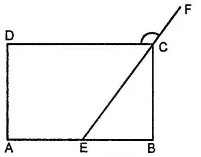State whether True or False.

(a) All rectangles are squares.

(b) All rhombuses are parallelograms.

(c) All squares are rhombuses and also rectangles.

(d) All squares are not parallelograms.

(e) All kites are rhombuses.

(f) All rhombuses are kites.

(g) All parallelograms are trapeziums.

(h) All squares are trapeziums.

Asked by Aaryan | 1 year ago |  172

##### Solution :-

(a) False. All squares are rectangles but all rectangles are not squares.

(b) True. Opposite sides of a rhombus are equal and parallel to each other.

(c) True. All squares are rhombuses as all sides of a square are of equal lengths. All squares are also rectangles as each internal angle measures 90°.

(d) False. All squares are parallelograms as opposite sides are equal and parallel.

(e) False. A kite does not have all sides of the same length.

(f) True. A rhombus also has two distinct consecutive pairs of sides of equal length.

(g) True. All parallelograms have a pair of parallel sides.

(h) True. All squares have a pair of parallel sides.

Answered by Sakshi | 1 year ago

### Related Questions

#### In the given figure, PQRS is a kite. Find the values of x and y.

In the given figure, PQRS is a kite. Find the values of x and y.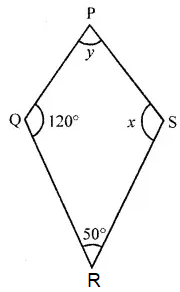#### In the given isosceles trapezium ABCD, ∠C = 102°. Find all the remaining angles of the trapezium.

In the given isosceles trapezium ABCD, ∠C = 102°. Find all the remaining angles of the trapezium.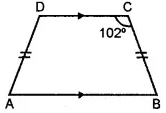#### In the given figure, ABCD is a rhombus and ∠ABD = 50°. Find :

In the given figure, ABCD is a rhombus and ∠ABD = 50°. Find :

(i) ∠CAB

(ii) ∠BCD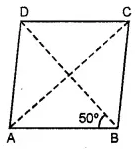#### In the given figure, ABCD is a rectangle and diagonals intersect at O. If ∠AOB = 118°, find

In the given figure, ABCD is a rectangle and diagonals intersect at O. If ∠AOB = 118°, find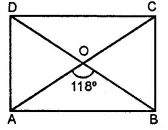(i) ∠ABO

(iii) ∠OCB